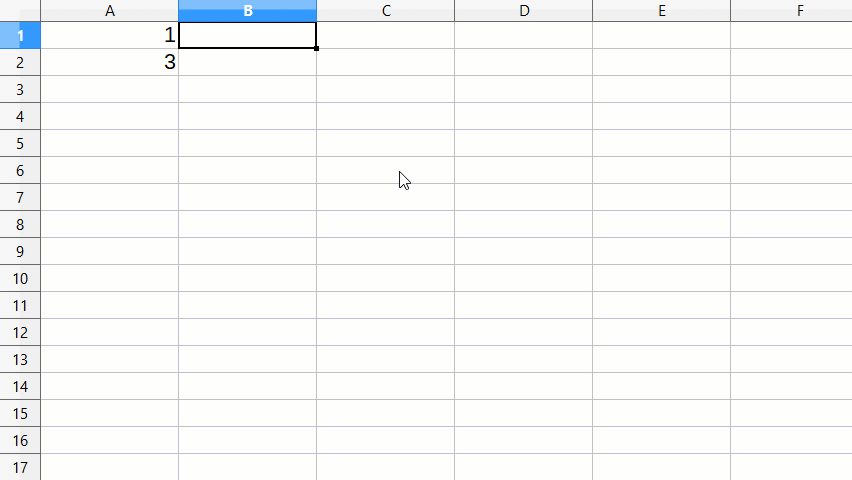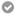# Smartly auto-increment called cells

When I try to increment called rows or columns in a sequence that skips one or sevral numbers such as:

``````=A1
=A3
=A5
=A7
=A9
``````

by providing the first 2 cells (e.g. =A1 and =A3), it doesn’t work.

``````=A1
=A3
=A3
=A5
=A5
=A7
=A7
=A9
=A9
``````

and it gets weirder if I try to help it further by providing the first 3 cells:

``````=A1
=A3
=A5
=A4
=A6
=A8
=A7
=A9
=A11
``````

Can I fix this ?

No - because you want A2=A1+2; A3=A2+2, … so you make useless use of ROW() function, which btw. works as expected. In your “2 cells” example:

• 1st value: take the number of current row
• 2nd value: take the number of next row

and these two rules are repeated if you drag down. Same applies for your “3 cells” example.

You had some reasonable formulas there (`=ROW(A1)`, `=ROW(A3)`); now you changed that to something that just couldn’t work if you put that into A1 and A2: putting there `=A1` and `=A3` would create cycles.

No because I actually want to increment the called cells, so I edited my post to better fit my problem. Sorry for my lack of clarity.

Boah - this should have been mentioned that you want increment cell references and not cell values.

Of course you get it. You have a sequence of two formulas, first reading “give me the number of current row”, the second one being “give me number of the row just below this”. You then copy the two formulas, and they continue this sequence: “this-row-#; next-row-#; this-row-#; next-row-#; this-row-#; …”.

Why don’t you just put plain numbers 1 and 3 into A1 and A2, and drag-copy that?

Because my situation involves formulas using several called cells which I need to increment smartly both vertically and horizontally. Splitting the formulas in plain text and incrementing the cell numbers & letters in separate rows or columns is impossible. I would need a 3rd dimension.

Then your formula would look like `=ROW()*2-1`. That’s it. It’s just a basic formula mapping all positive integers into all positive odd numbers.
You need to come with other formulas for possible other sequences.

Another way: put `1` into A1; put `=A1+2` into A2; drag-copy A2.

Thanks but that’s not what I mean. What you propose increments the output value. What I want is to properly increment the called cells. Imagine my formulas have to take values of one in 4 rows and one in 3 columns.
Sorry for my lack of clarity. I edited the original post, which should be clearer now.

Sigh. Could you at least mention which column are you showing us? I was under an impression that we are talking about column A.

Put `=OFFSET(A1;ROW()-1;0)` into B1 and drag-copy to get reference to every second row in column A.

Or, if you like:

``=OFFSET(\$A\$1;(ROW()-1)*2;0)``

Actually, it’s easy:If my answer has solved your problem, please click on the checkmark ✓ in the circleto the left of the answer and click on the arrow ^ for upvote. This will tell the community that the question has been answered correctly.

With me Windows 10 Home; Version 1903; 64-Bit | LibreOffice, Version: 6.2.4.2 (x64).